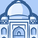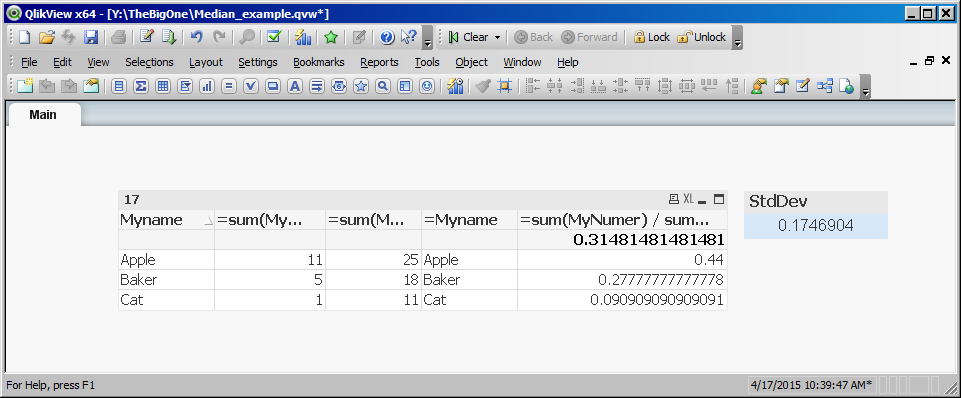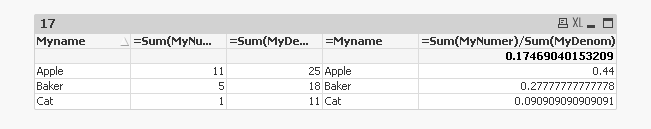# QlikView App Dev

Discussion Board for collaboration related to QlikView App Development.

Announcements
Join “Do More With Qlik” with Mike Tarallo to get a first-hand look at the New Authoring Experience, June 29th: REGISTER HERE
cancel
Showing results for
Did you mean:Contributor III

## problem with stddev and median

Here is the problem:

I need to use both the standard deviation and the median function.

My data has Numerators and Denominators. Below is what an example table might look like.

The problem is that all the numerators for a single name (Myname) need to be summed.

Then

All the Denominators for a single name (MyName) need to be summed. This sum must be performed before I can do the division i.e. Numerator Over Denominator. I can get the standard deviation by creating a chart and using the StdDev total as the column summary, but I need to do this in a text box. Additionally the Median function is not available in the Chart column total.

I thought I could do this as follows:

=Stdev (aggr(sum(MyNumer),Myname) / aggr(sum(MyDenom),Myname))

But the answer I get is not correct. How do I know? because I compare it to the stddev function in the chart as described above.

Here is an screen shot of the data in the table I enclosed. Notice the chart gives a standard deviation of .3148 While my text box with the above formula gives 0.17

Is there a way to do this ?

TerenceTable:
[
MyMonth, Myname, MyNumer, MyDenom
1, Apple, 1, 10
2, Apple, 1, 2
3, Apple, 3, 4
4, Baker, 1, 5
5, Baker, 2, 5
6, Baker, 1, 7
7, Apple, 4, 5
8, Cat, 0, 1
9, Cat, 1, 10
10, Baker, 1, 1
11, Baker, 0, 0
12 ,Apple, 2, 4
]
;

4 RepliesMVP

I am getting a different number for st dev in the chart object (equal to what you have for the text box object). Have a look:Also attaching the application (PFA)

Best,

SunnyMVP

For median you can do something like this as your chart expression:

=Median(Aggr(Sum(MyNumer)/Sum(MyDenom), Myname))

HTH

Best,

SunnyContributor III
Author

Wait one second.

Let's forget the median issue and just focus on Standard deviation.

If you look at my example, you will see that the stddev which is generated via Qlik in the chart, is the correct standard deviation.

The point is, I need this number in a text box. I could pull the number off the Chars via a Macro, but I don't want to use a Macro because they don't work in a webb base. Therefore I want to use a calculation in a text box. I thought the formula/calculation I stated in the original post would work, but it yields a different number than that of the the Qlik Chart generated StdDev.

Additionally, If you attempt the same formula as I am using in the text box with Median instead of StdDev, you will also find that the numbers do not match.

Essentially, the text box formula does not work, and I don't know why.

Does any one have a different formula I can use which would yield the correct number ?

terenceMVP

Can you share the sample qvw you are working with?

Best,

Sunny